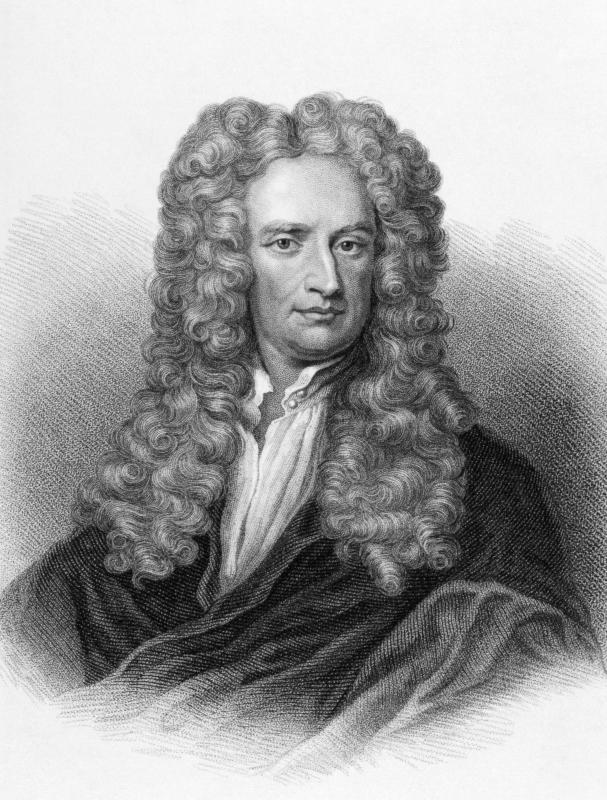# What Is Tangential Acceleration?

M.J. Casey
M.J. CaseyIsaac Newton derived tangential acceleration in the 1600s.

Many objects travel in a circular motion. These include ice skaters, cars, and planets. In the late 1600s, Isaac Newton studied circular motion and defined several new properties of these systems. Tangential acceleration is one of the components he derived, among many others.

Newton observed that an object once in motion will travel in a straight line unless an external force is applied. An object traveling a circular path is subject to a force that is either pulling or pushing toward the center of the circle, called the normal or centripetal force. Neither of these forces is along the curved path. They are continuously at right angles to each other.

In linear motion, an object once set in motion will stay in motion unless acted upon by another force. Additional energy is not required. This is not true of circular motion.

The object moving in a circle at a constant rate, measured in revolutions per minute, has a constant tangential velocity and a constant angular velocity. In linear motion, when the velocity is constant, acceleration is zero. The tangential acceleration is positive. Energy is required to keep changing direction continuously.

Tangential acceleration is equal to tangential velocity squared, divided by the radius. It is also calculated by the radius times the angular velocity squared. Two observations can be made about tangential acceleration from these equations. Linear acceleration is a factor of only velocity, while tangential acceleration is a factor of velocity squared. The sensation of speed is much stronger in a turning car than one that is moving at the same linear velocity in a linear direction.

The tangential acceleration is a factor of the radius. As the radius gets larger, the tangential acceleration gets smaller for the same angular velocity. Stated differently, as the radius gets smaller, with no additional energy input, the angular velocity increases.

People take advantage of the laws of motion as applied to circular or curved paths on a daily basis. Skilled drivers slow down first and then keep the gas pedal slightly engaged during tight turns. The additional energy keeps the wheels rolling forward instead of skidding sideways.

Skidding occurs when the centripetal force that feeds the tangential acceleration decreases. Ice skaters tuck their arms and free leg close to their body to spin faster. Several space missions have used the gravitational pull of the moon or other celestial bodies to accelerate the space capsule into a desired curved path.

## You might also Like## Discuss this Article

Forgot password?
###### Register:
•Isaac Newton derived tangential acceleration in the 1600s.